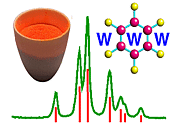Orthorhombic Space Groups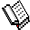Course Material Index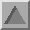Section Index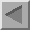Previous Page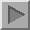Next Page

Orthorhombic Space Groups

There are 2 triclinic, 13 monoclinic, and 59 orthorhombic space groups. Given the large number involved, it is clearly important to learn some general properties of orthorhombic space groups. The orthorhombic space groups are best divided into three groups based on crystal class: those that contain just 2 or 21 axes (class 222), those that contain a single 2 or 21 axes plus two mutually perpendicular planes (class mm2), and those that are centrosymmetric with both 2 or 21 axes and three mutually perpendicular planes (class mmm). Orthorhombic space groups belonging to the crystal class 222 are enantiomorphic, while those belonging to the crystal class mm2 are polar. The result of the mutually perpendicular axes and/or planes is to constrain all of the unit cell angles to 90°, i.e. the unit cell axes are orthogonal to each other, but without constraint on their magnitude.

The large number of orthorhombic space groups arises from the large number of ways in which twofold rotation and two-one screw axes and mirror and glide planes can interact together in three dimensions. If one considers the case of a primitive orthorhombic lattice with only twofold or two-one screw axes, then 4 unique combinations can be found corresponding to the space groups: P222, P2221, P21212, and P212121. These space groups are all characterised by the presence of twofold rotation or two-one screw axes parallel to each of the unit cell directions a, b, and c.

The space group symbol for the orthorhombic space groups is composed of the lattice centring followed by the symmetry with respect to the a, b, and c-axes directions. Thus, for example, the space group P2221 has twofold rotation axes parallel to both a and b plus a two-one screw axis parallel to c.

Although the space groups P222, P2221, P21212, and P212121 obviously differ in the number of twofold rotation and two-one screw axes that each possesses, they also differ in how these symmetry elements interact with each other in three-dimensional space. In space group P222, the three twofold rotation axes all intersect at a single point, which is chosen as the origin for this space group. By contrast, in space group P212121 none of the two-one screw axes intersect at all.

To illustrate some points concerning orthorhombic space groups, we will consider the space group Pnma, which is shown in the figure below: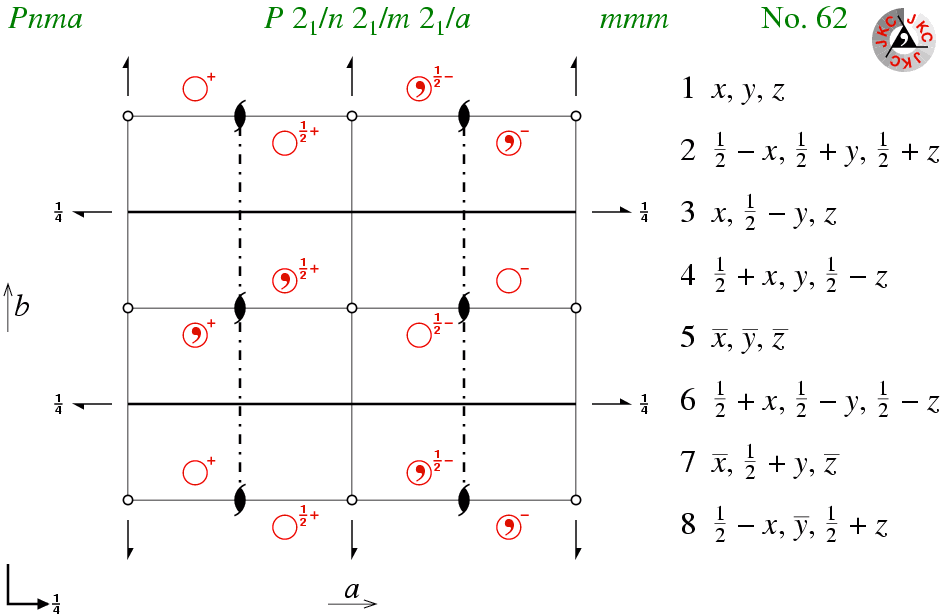You will see from long space-group symbol that this orthorhombic space group possesses three mutually perpendicular two-one screw axes. As in the space group P212121, none of the screw axes intersect in space. The origin for this space group has been chosen to coincide with a point of inversion. Note that the origin in this space group is equidistant from each of the 3 two-one screw axes, i.e. at 1/4 of a unit cell length in a, b, and c from each screw axis. In the clickable figure that follows this page, you will see that the origin of space group P212121 is chosen likewise: space group P212121 is a subgroup of space group Pnma (and Pnma is a supergroup of P212121), the common origin emphasising the sub-supergroup relationship between the two.

Pnma is centrosymmetric with 8 points of inversion per unit cell. Along each of the unit-cell directions, there are 4 two-one screw axes per unit cell (i.e. a total of 12), and perpendicular to each of the unit cell axes there are two planes per unit cell (i.e. a total of 6). The thick solid line shows the position of the two mirror planes, while the dash-dotted line shows the location of the two n-glide planes within the unit cell; dashes and dots are used to indicate that the glide component is both parallel to the line and perpendicular to it. This demonstrates that the concepts learnt using space groups P-1 and P21/c are equally valid here too. Also observe that, like the two-one axes, none of the planes pass through the origin for this particular space group.

This space group was listed in the top 10 of most frequently-observed space groups for molecular crystals. You may be surprised by this given that the mirror plane does not normally favour close packing of molecules. However, where a molecule has mirror plane symmetry, then this type of space group will favour close packing since the only other symmetry elements are either screw axes or glide planes. The primitive centrosymmetric orthorhombic space group with only screw axes and glide planes is Pbca; not surpisingly this is also a commonly-observed space group, as listed earlier.

When you have tried the clickable figure that follows, return to this space group and see if you can identify how the symmetry-equivalent points within the unit cell are related to the initial point in the bottom left-hand corner of the diagram.Course Material IndexSection IndexPrevious PageNext Page
 © Copyright 1995-2006.  Birkbeck College, University of London. Author(s): Jeremy Karl Cockcroft Huub Driessen